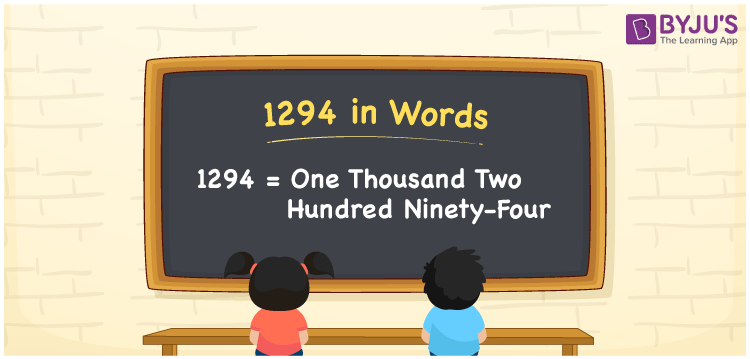# 1294 in Words

The number name of 1294 is expressed as one thousand two hundred ninety-four. In mathematics, 1294 is a cardinal number. For example, the cost of 2 flower bouquets is Rs. 1294. In this article, we are going to learn the procedure of writing the number 1294 in words using the place value system in detail.

 1294 in Words: One thousand two hundred ninety-four. One thousand two hundred ninety-four in Numerical Form: 1294.

## 1294 in English Words## How to Write 1294 in Words?

The place value table for the numeral 1294 is provided below:

 Thousands Hundreds Tens Ones 1 2 9 4

The expanded form of 1294 is as follows:

= 1 × Thousand + 2 × Hundred + 9 × Ten + 4 × One

= 1 × 1000 + 2 × 100 + 9 × 10 + 4 × 1

= 1000 + 200 + 90 + 4

= 1294

= One thousand two hundred ninety-four

Hence, 1294 in words is one thousand two hundred ninety-four.

1294 in words – One thousand two hundred ninety-four

Is 1294 an odd number? – No

Is 1294 an even number? – Yes

Is 1294 a perfect square number? – No

Is 1294 a perfect cube number? – No

Is 1294 a prime number? – No

Is 1294 a composite number? – Yes

## Frequently Asked Questions on 1294 in Words

Q1

### How to spell 1294 in English words?

1294 in words is one thousand two hundred ninety-four.

Q2

### Simplify 1200 + 94, and express it in words.

Simplifying 1200 + 94, we get 1294. Hence, 1294 in words is one thousand two hundred ninety-four.

Q3

### How to write one thousand two hundred ninety-four in numbers?

One thousand two hundred ninety-four in numbers is 1294.